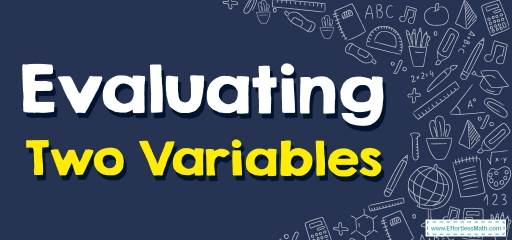# How to Evaluate Two Variables? (+FREE Worksheet!)

You can evaluate a mathematical expression with two variables when you have the values of the variables.## Step by step guide to evaluating two variables

• To evaluate an algebraic expression, substitute a number for each variable and perform the arithmetic operations.

### Evaluating Two Variables – Example 1:

Solve this expression. $$4 \ (2a \ – \ b), \ a=2, \ b=\ – 1$$

Solution:

First use distributive property formula: $$?(? + ?)=?? + ??$$
$$4 \ (2a \ – \ b)= 8a \ – \ 4b$$

Now, substitute $$2$$ for $$a$$ , and $$- \ 1$$ for $$b$$, then:
$$4 \ (2a \ – \ b)= 8a \ – \ 4b= 8 \ (2) \ – \ 4 \ (- 1)=16 \ + \ 4=20$$

### Evaluating Two Variables – Example 2:

Solve this expression. $$2x \ + \ 6y, x=1 \ , \ y=2$$

Solution:

First substitute $$1$$ for $$x$$, and $$2$$ for $$y$$ , then:
$$2x \ + \ 6 y =2 \ (1) \ + \ 6 \ (2)=2 \ + \ 12=14$$

### Evaluating Two Variables – Example 3:

Solve this expression. $$-3x+5y ,x=2,y=-1$$

Solution:

First substitute $$2$$ for $$x$$, and $$-1$$ for $$y$$, then:
$$-3x+5y=-3(2)+5(-1)=-6-5=-11$$

### Evaluating Two Variables – Example 4:

Solve this expression. $$2(a-2b),a=-1,b=3$$

Solution:

First use distributive property formula: $$?(? + ?)=?? + ??$$

$$2(a-2b)=2a-4b$$

Now, substitute $$-1$$ for $$a$$ , and $$3$$ for $$b$$ , then:
$$2(a-2b)=2a-4b=2(-1)-4(3)= -2-12= -14$$

## Exercises for Evaluating Two Variables

### Simplify each algebraic expression.

• $$\color{blue}{2x + 4y – 3 + 2, \\ x = 5, y = 3 } \\$$
• $$\color{blue}{(– \frac{12}{x}) + 1 + 5y, \\ x = 6, y = 8} \\$$
• $$\color{blue}{(– 4) (– 2a – 2b), \\ a = 5, b = 3} \\$$
• $$\color{blue}{10 + 3x + 7 – 2y, \\ x = 7, y = 6} \\$$
• $$\color{blue}{9x + 2 – 4y, \\ x = 7, y = 5} \\$$
• $$\color{blue}{6 + 3 (– 2x – 3y), \\ x = 9, y = 7} \\$$

• $$\color{blue}{21}$$
• $$\color{blue}{39}$$
• $$\color{blue}{64}$$
• $$\color{blue}{26}$$
• $$\color{blue}{45}$$
• $$\color{blue}{-111}$$

### What people say about "How to Evaluate Two Variables? (+FREE Worksheet!) - Effortless Math: We Help Students Learn to LOVE Mathematics"?

No one replied yet.

X
30% OFF

Limited time only!

Save Over 30%

SAVE $5 It was$16.99 now it is \$11.99